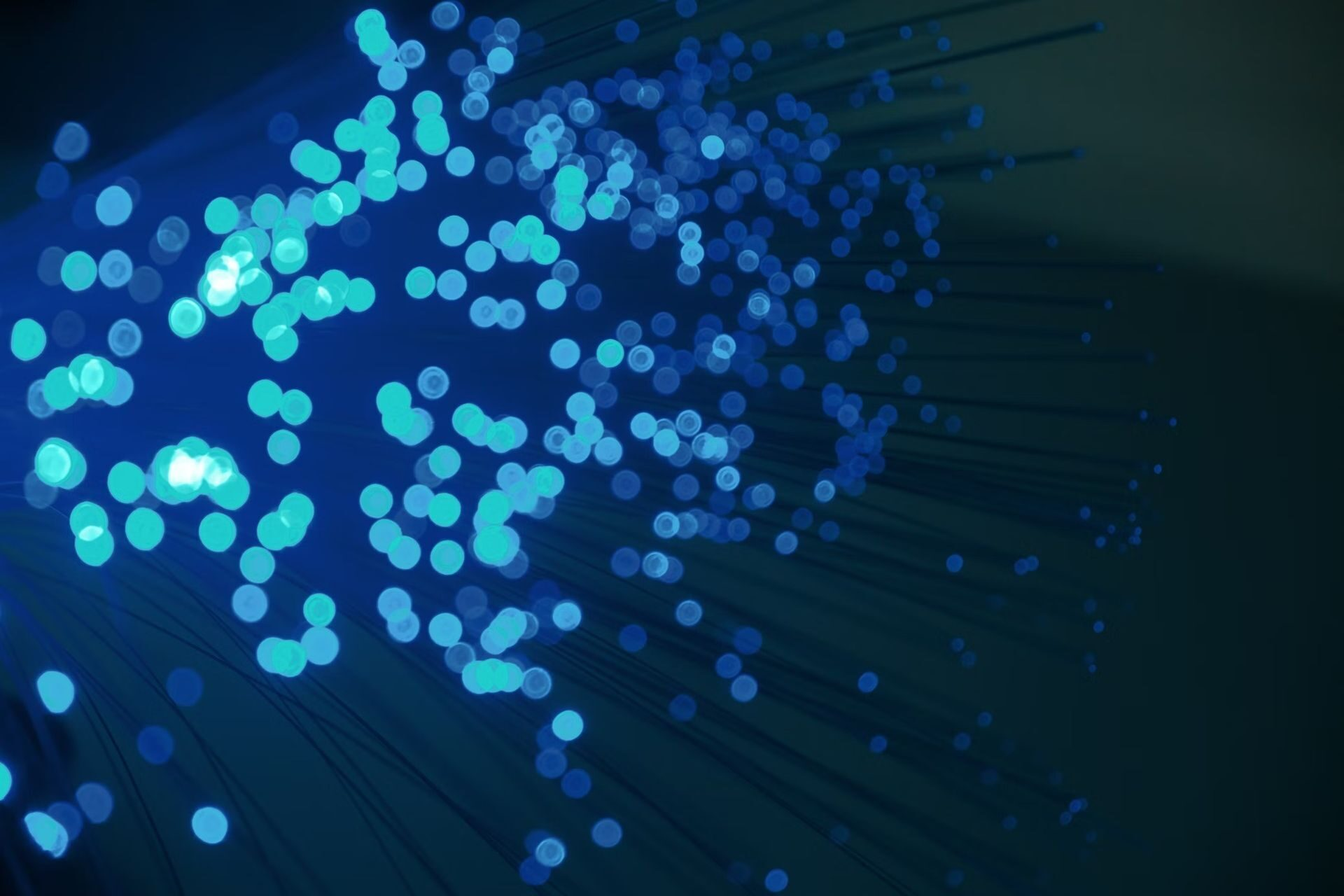Opposable thumbs have played an essential role in the evolution of our species. However, if evolution had given us two additional thumbs, things would not be much different. A single thumb on each hand is sufficient.

But not for neural networks, the most sophisticated AI technologies for performing human-like activities, as they’ve grown bigger, they’ve been able to grasp more information. This has come as a surprise to spectators. Basic mathematical findings had predicted that networks should only require so much space, but modern neural networks are frequently expanded far beyond this theoretical limit – known as overparameterization. Did you know, neural network-based visual stimuli classification paves the way for early Alzheimer’s diagnosis? So these kind of researches are really important for neural network systems to improve.

## The latest study proved that neural networks must be bigger

In December, Microsoft Research’s Sébastien Bubeck and  Mark Sellke from Stanford University offered a new theory for the scaling problem in a paper presented at NeurIPS, a major conference. They argue that neural networks must be considerably bigger than previously thought to avoid several basic issues. The discovery provides a broader understanding of an issue that has perplexed experts for decades.

“It’s a really interesting math and theory result. They prove it in this very generic way. So in that sense, it’s going to the core of computer science,” said the Swiss Federal Institute of Technology Lausanne, Lenka Zdeborová.The typical standards for the size of neural networks are based on studies of how they memorize data.

The typical standards for the size of neural networks are based on studies of how they memorize data. However, to understand memory, we must first grasp what networks accomplish.

The identification of objects in photographs is a typical operation for neural networks. To create a network that can do this, researchers first give it many photos and object labels, instructing it to discover the relationships between them. The network will correctly identify the item in a photo previously seen after being trained. A network learns data when it is trained. In addition, once a network has memorized enough training data, it also achieves the capacity to forecast the labels of objects that it has never seen — to some extent—the ability for a network to generalize.

The amount of information a neural network can retain is determined by its size. This may be pictured as follows. Assume you have two data points from which to choose. You can link these points with a line with two parameters: the slope and the height when it crosses the vertical axis. Suppose someone else is given the line, as well as an x-coordinate of one of the original data points. In that case, they may deduce the corresponding y-coordinate simply by looking at it (or using the parameters). The line has memorized the two data points.

Similar things may be said of neural networks. Images, for example, are described by hundreds or thousands of numerical values — one for each pixel. This set of numerous free parameters is mathematically equivalent to a point’s position in a high-dimensional space. The dimension (number of coordinates) is known as the dimension.

An old mathematical result says that to fit n data points with a curve, you need a function with n parameters. (In the previous example, the two points were described by a curve with two parameters.) When neural networks first emerged as a force in the 1980s, it made sense to think the same. They should only need n parameters to fit data points — regardless of the dimension of the data.

To accommodate n data points with a curve, it is necessary to use a function with n parameters, according to an old mathematical theorem. (In the previous example, the two points were represented by a curve with two parameters.) It made perfect sense when neural networks first emerged in the 1980s. There should only be n parameters required to fit n data points, regardless of the data size.

“This is no longer what’s happening. Right now, we are routinely creating neural networks that have a number of parameters more than the number of training samples. This says that the books have to be rewritten,” explained Alex Dimakis of the University of Texas, Austin.

Bubeck and Sellke weren’t attempting to rewrite anything when they decided to tackle the problem. They were examining a different feature that neural networks frequently lack, robustness, which is the ability of a network to deal with minor variations. A network that isn’t robust may have learnt to recognize a giraffe, but it would mistake an extremely modified variant for a gerbil. When Bubeck et al realized in 2019 that the problem was linked to network size, they set out to disprove theorems about it.

“We were studying adversarial examples — and then scale imposed itself on us. We recognized it was this incredible opportunity, because there was this need to understand scale itself,” explained Bubeck.

In their new proof, the pair demonstrates that robustness requires overparameterization. They calculate how many parameters are required to properly fit data points to a curve with a mathematical quality equivalent to smoothness.

Consider a plane curve, with the x-coordinate representing the color of a single-pixel and the y-coordinate representing an image label. Because the curve is smooth, the prediction should only change modestly if you move a little distance along it while changing the pixel’s color slightly. However, because an extremely sharp curve has been described, small changes to the x-axis (the color) can produce huge differences in the y-coordinate (the image label). Giraffes may turn into gerbils.